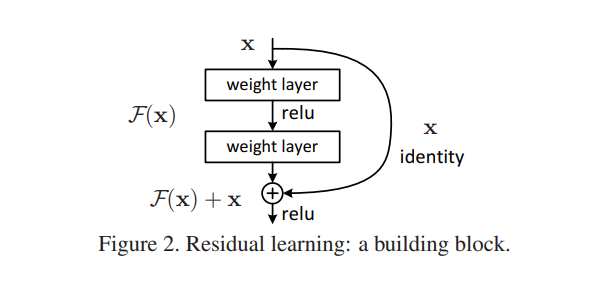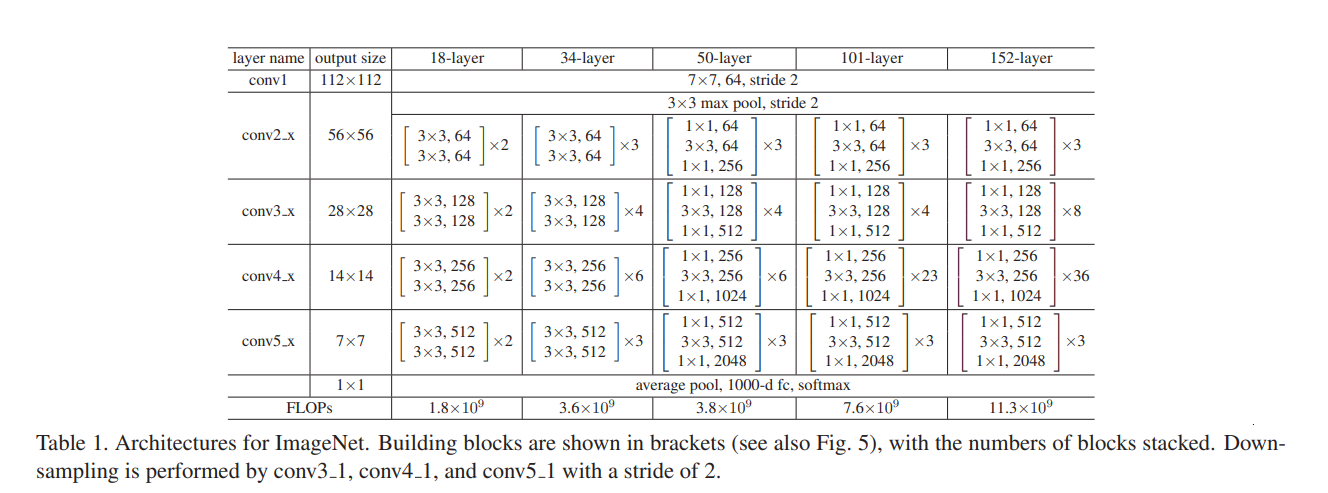# Residual Network: Code Explained

In a nutshell, Residual Network(ResNet) is a type of deep learning architecture that recast the input and the output of the stacked layer. Deep Residual Network was introduced in the paper called Deep Residual Network for Image Recognition published in 2016 and experimented using ImageNet dataset and won the challenge in the first position. Not only that, the paper also examine the other dataset such as CIFAR-10, PASCAL, and MS COCO. Object detection task was also examined and put the ResNet as the backbone network.He et. al. Deep Residual Network for Image Classification. CVPR, 2016.

But, when I read about this in 2018, and try to understand the idea of the residual learning, I did understood. But, not in the implementation. So, I tried to dive into the code and I found TorchVision that has this architecture. This article explains the torchvision the model code.He et. al. Deep Residual Network for Image Classification. CVPR, 2016.

If you aren’t familiar with PyTorch, you can read a short brief here and the code of ResNet can be found here. For the sake of example, ResNet18 will be used to understand how it works.

What made me confused earlier when I read about ResNet was that, the input feeds into convolution network and the output adds the input which doesn’t make sense because usually the input and the output have different shapes. First, I thought maybe they uses padding in the convolution so the have the same shape and I started to test the convolution configuration used in the torchvision.

Here is the convolution function in torchvision

Which then generates the network

Because of padding on all sides, the input and the output is the same

The padding makes the the recast possible. So then, we just make the entire blocks.

So yeah, that’s it.

--

--